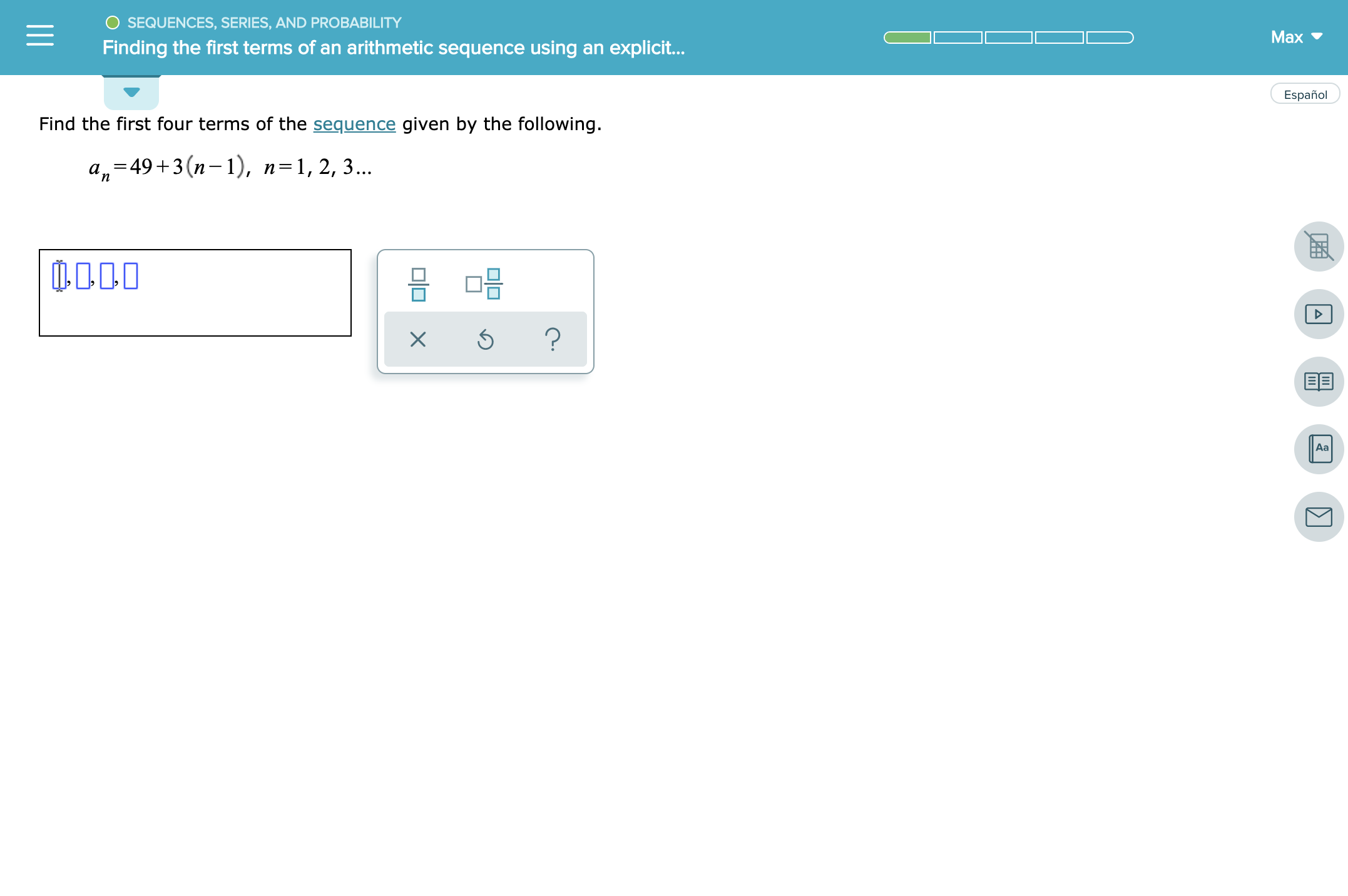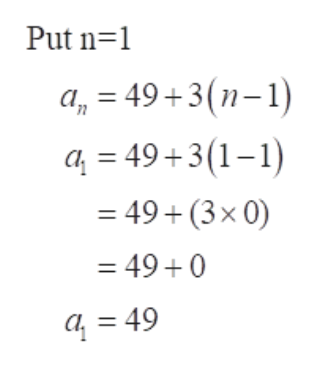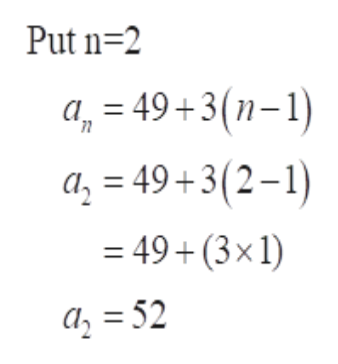# OSEQUENCES, SERIES, AND PROBABILITYMaxFinding the first terms of an arithmetic sequence using an explicit...EspañolFind the first four terms of the sequence given by the following.a-49+3 (n-1), n=1, 2, 3...0000?XAaOOII

Question
3 views

see attachedhelp_outlineImage TranscriptioncloseOSEQUENCES, SERIES, AND PROBABILITY Max Finding the first terms of an arithmetic sequence using an explicit... Español Find the first four terms of the sequence given by the following. a-49+3 (n-1), n=1, 2, 3... 0000 ? X Aa OO II fullscreen
check_circle

Step 1

To find the first four terms of the given sequence substitute the value of n = 1, 2, 3 & 4 and get the value of a1, a2, a3 & a4.

Step 2

Put the value of n = 1help_outlineImage TranscriptionclosePut n 1 an =49+3(n-1) a = 49+3(1-1) 49+ (3x 0) = 49 + 0 = 49 fullscreen
Step 3

Put the value of n = 2...help_outlineImage TranscriptionclosePut n 2 a, 49+3(n-1) a, - 49+3(2-1) = 49 + (3x 1) = 52 a fullscreen

### Want to see the full answer?

See Solution

#### Want to see this answer and more?

Solutions are written by subject experts who are available 24/7. Questions are typically answered within 1 hour.*

See Solution
*Response times may vary by subject and question.
Tagged in

### Other